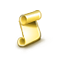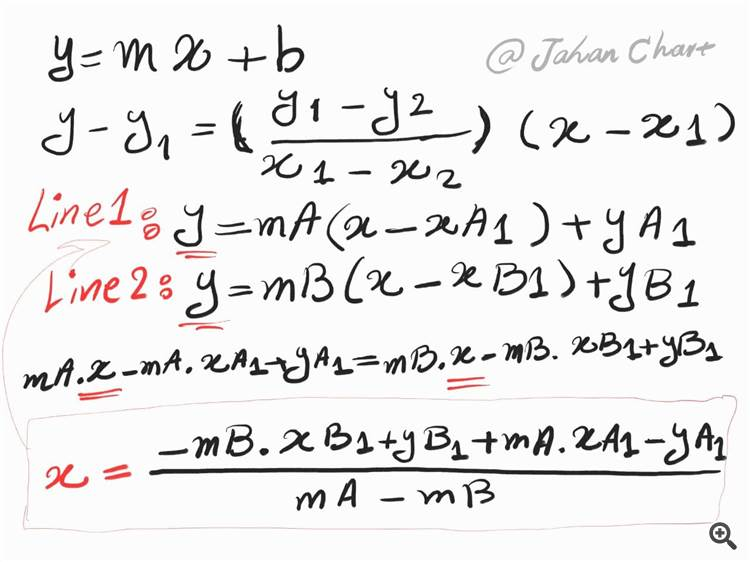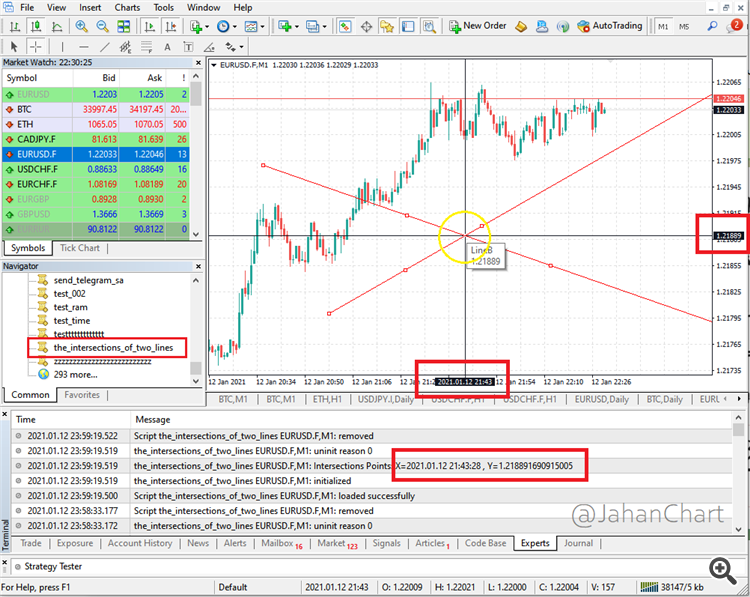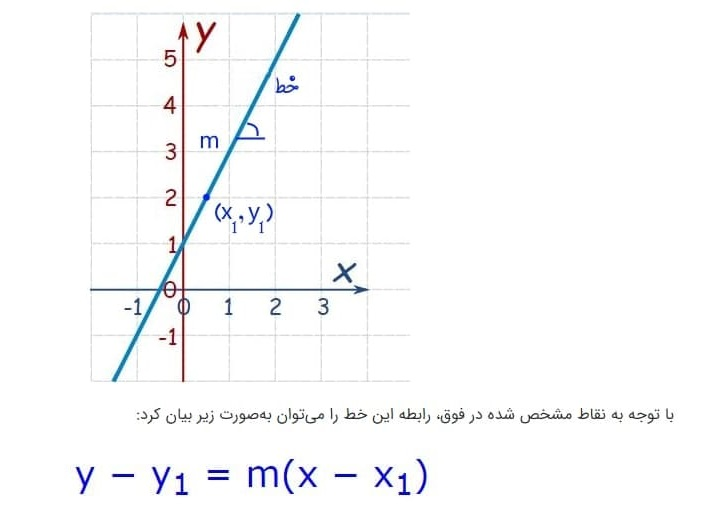Interesting script?
So post a link to it -
let others appraise it
You liked the script? Try it in the MetaTrader 5 terminal# The intersections of two lines - script for MetaTrader 4

Views:
1005
Rating:
Published:
2021.01.12 23:32

A friend asked a question, which became an excuse for me to play some memory with the math lessons of years ago! 😊 (Then say what is the use of high school arithmetic and geometry; this is a very practical example of the same course 😉)

In MetaTrader 4, we intend to calculate the intersection coordinates of two straight line objects with different slopes using MQL4.

I will give a general explanation:

If the horizontal axis of the chart means time X and the vertical axis of the chart means price Y. For each line on the chart having two points from it or one point and the slope of the line, get the equation of those two lines.

Finally we have a set of equations, which, by mathematical calculations and writing one variable on another and substituting it for another function, gives the values ​​of X and Y, which are the coordinates of the intersection of two lines.

I tried to solve the problem with a few simple and clean lines of code, which also has an educational aspect, I also put down the source code file.Telegram: @JahanChartSend a message from metatrader to telegram

With this script, you can send a message from MetaTrader 4 to Telegram.Smart Forex System Open Version

Force=((Bid-close)/close)*10000;Dashboard - Fenix CapitalMACD Sample